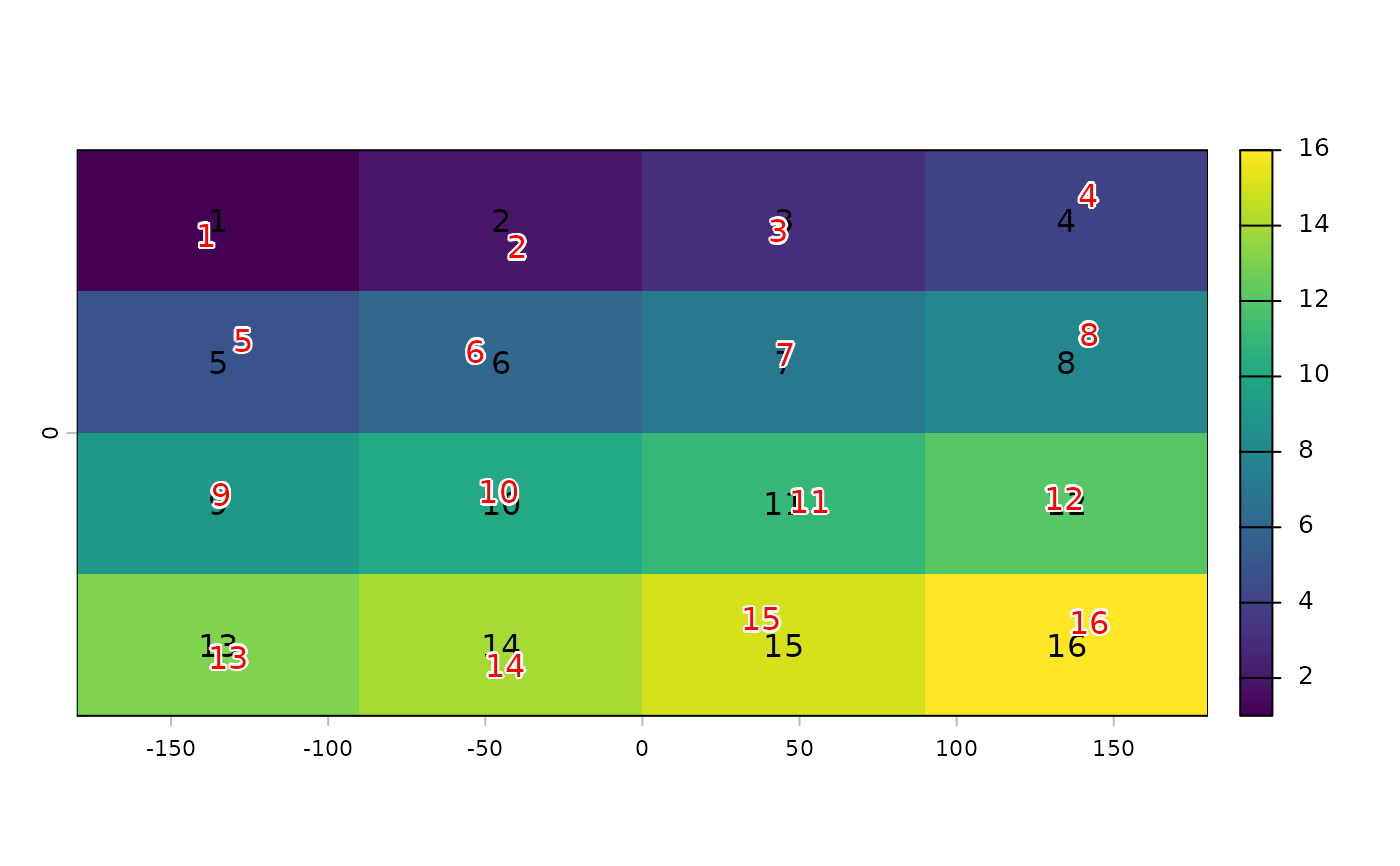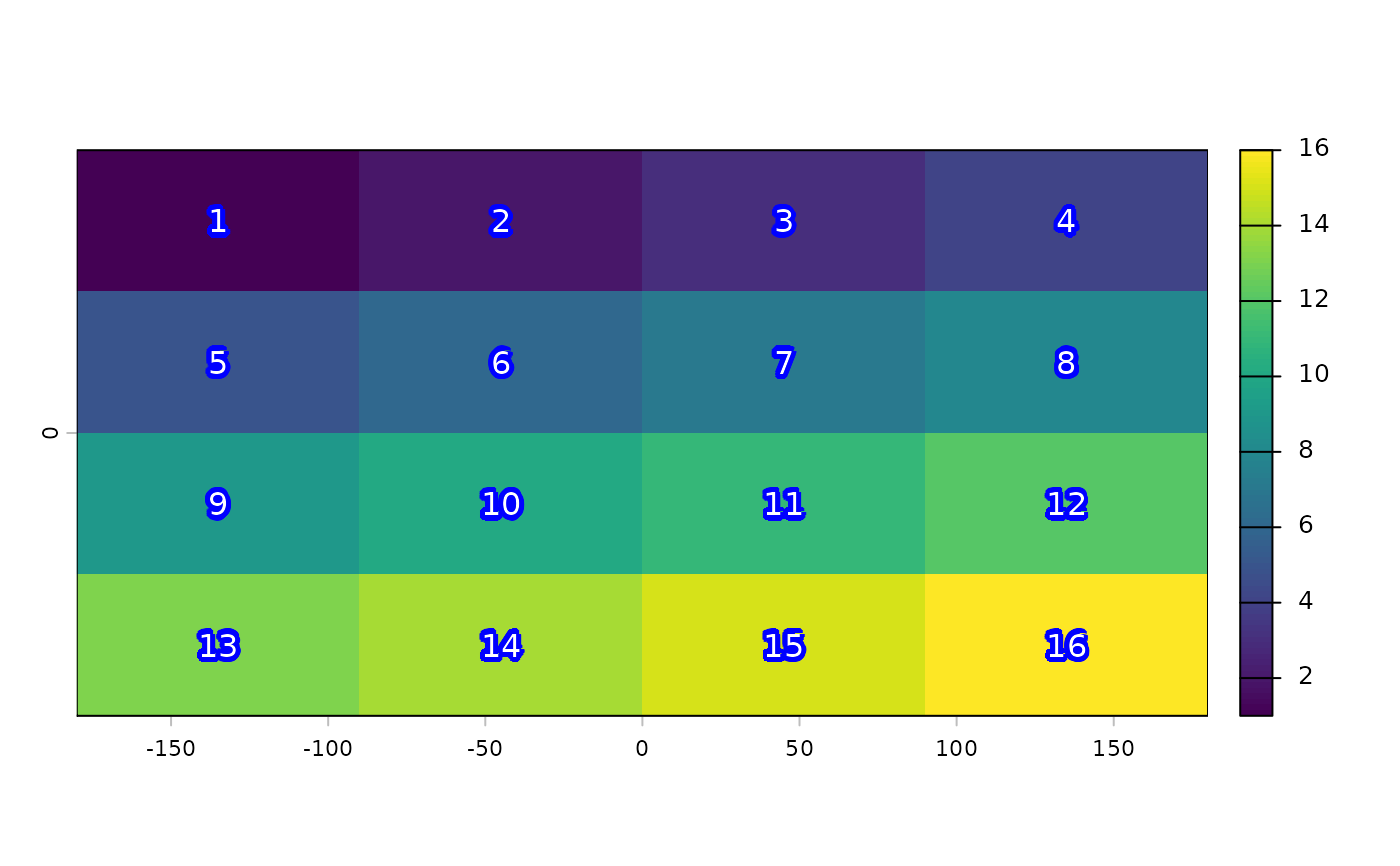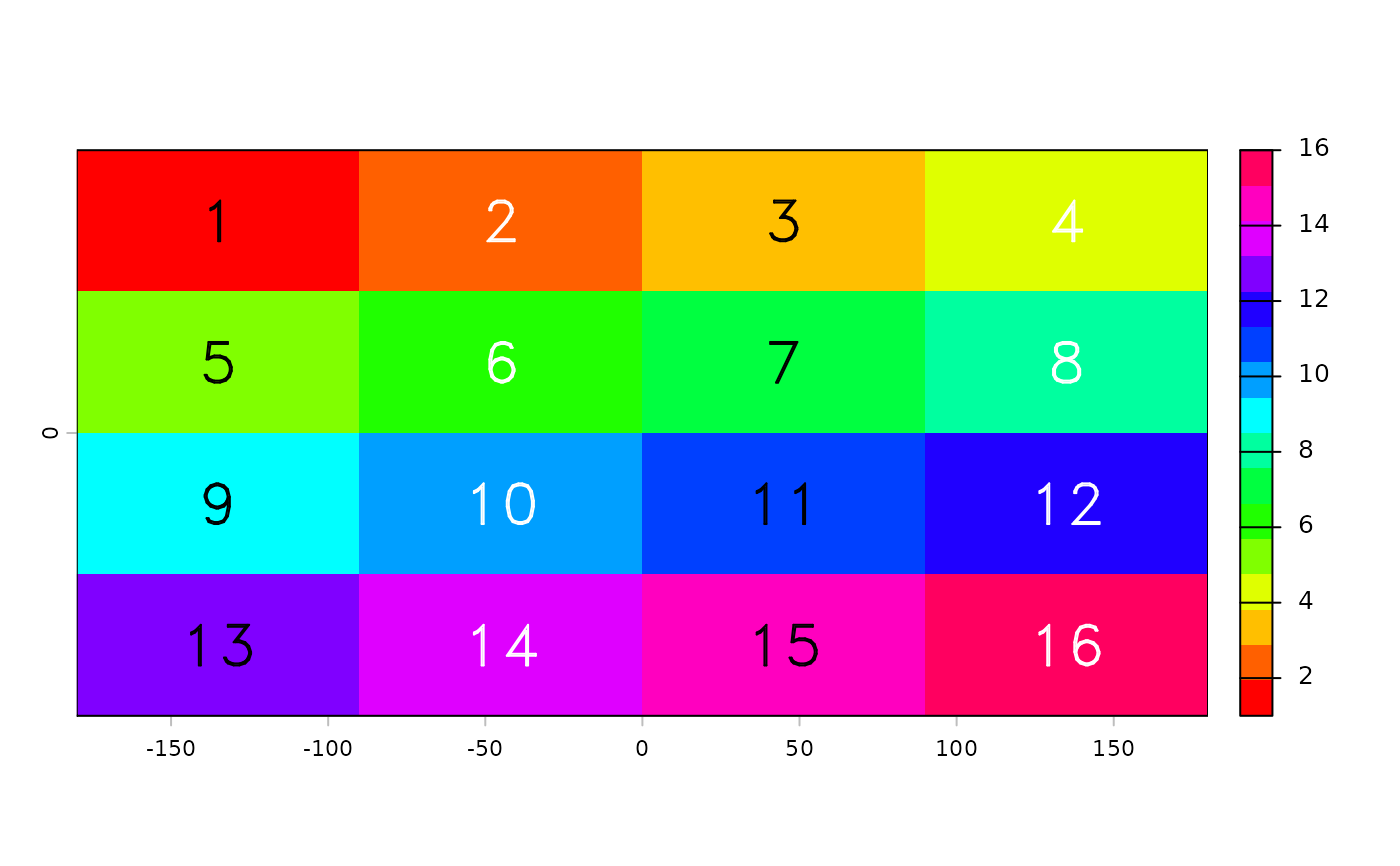Plots labels, that is a textual (rather than color) representation of values, on top an existing plot (map).

# S4 method for SpatRaster
text(x, labels, digits=0, halo=FALSE, ...)

# S4 method for SpatVector
text(x, labels, halo=FALSE, inside=FALSE, ...)

## Arguments

x

SpatRaster or SpatVector

labels

character. Optional. Vector of labels with length(x) or a variable name from names(x)

digits

integer. How many digits should be used?

halo

logical. If TRUE a "halo" is printed around the text. If TRUE, additional arguments hc="white" and hw=0.1 can be modified to set the color and width of the halo

inside

logical. Should the text always be placed inside one the sub-geometries?

...

additional arguments to pass to graphics function text

text, plot, halo

## Examples

r <- rast(nrows=4, ncols=4)
values(r) <- 1:ncell(r)
plot(r)
text(r)plot(r)
text(r, halo=TRUE, hc="blue", col="white", hw=0.2)plot(r, col=rainbow(16))
text(r, col=c("black", "white"), vfont=c("sans serif", "bold"), cex=2)